# Logged Sensor Data Alignment for Orientation Estimation

This example shows how to align and preprocess logged sensor data. This allows the fusion filters to perform orientation estimation as expected. The logged data was collected from an accelerometer and a gyroscope mounted on a ground vehicle.

Load logged inertial measurement unit (IMU) data and extract individual sensor data and timestamps.

```load('imuData', 'imuTT') time = imuTT.Time; accel = imuTT.LinearAcceleration; gyro = imuTT.AngularVelocity; orient = imuTT.Orientation; ```

### Inspect the Gyroscope Data

From the range of angular velocity readings, the logged gyroscope data is in radians per second instead of degrees per second. Also, the larger z-axis values and small x- and y-axis values indicate that the device rotated around the z-axis only.

```figure plot(time, gyro) title('Gyroscope') ylabel('rad/s') legend('x-axis', 'y-axis', 'z-axis') ```### Inspect the Accelerometer Data

Since the z-axis reading of the accelerometer is around 10, the logged data is in meters per second squared instead of g's.

```figure plot(time, accel) title('Accelerometer') ylabel('m/s^2') legend('x-axis', 'y-axis', 'z-axis') ```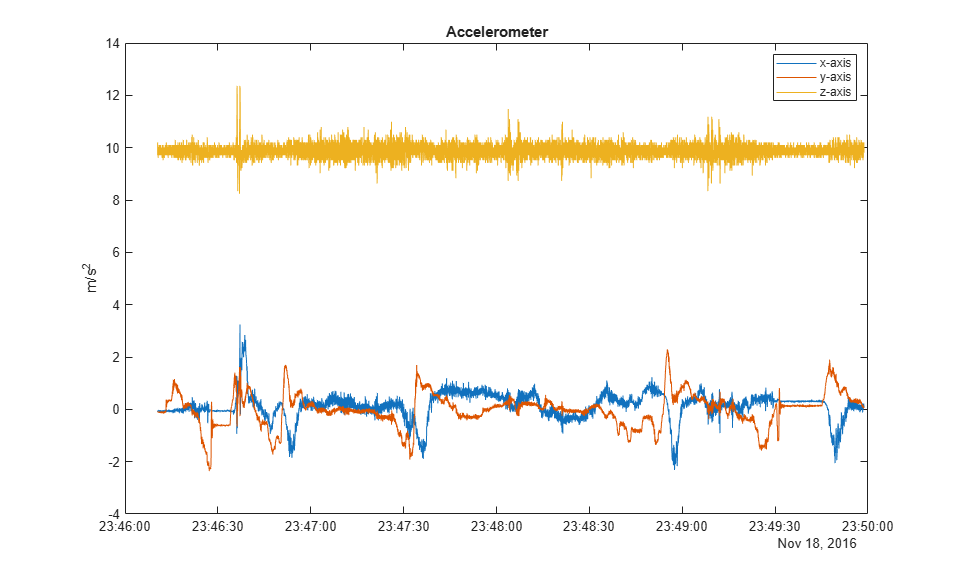### Inspect the Orientation Data

Convert the logged orientation quaternion data to Euler angles in degrees. The z-axis is changing while the x- and y-axis are relatively fixed. This matches the gyroscope and accelerometer readings. Therefore, no axis negating or rotating is required. However, the z-axis Euler angle is decreasing while the gyroscope reading is positive. This means that the logged orientation quaternion is expected to be applied as a point rotation operator (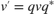). In order to have the orientation quaternion match the orientations filters, such as `imufilter`, the quaternion needs to be applied as a frame rotation operator (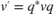). This can be done by conjugating the logged orientation quaternion.

```figure plot(time, eulerd(orient, 'ZYX', 'frame')) title('Euler Angles') ylabel('\circ') % Degrees symbol. legend('z-axis', 'y-axis', 'x-axis') ```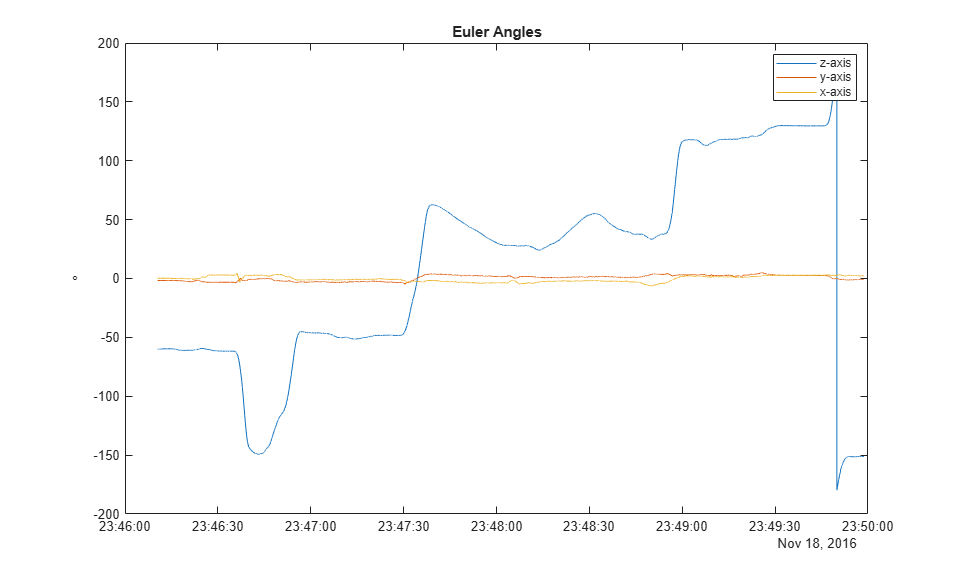### Find the Sampling Rate of the Logged Data

An estimate of the sampling rate can be obtained by taking the mean of the difference between the timestamps. Notice that there are some variances in the time differences. Since the variances are small for this logged data, the mean of the time differences can be used. Alternatively, the sensor data could be interpolated using the timestamps and equally spaced timestamps as query points.

```deltaTimes = seconds(diff(time)); sampleRate = 1/mean(deltaTimes); figure plot([deltaTimes, repmat(mean(deltaTimes), numel(deltaTimes), 1)]) title('Time Differences') ylabel('s') legend('differences', 'mean') ```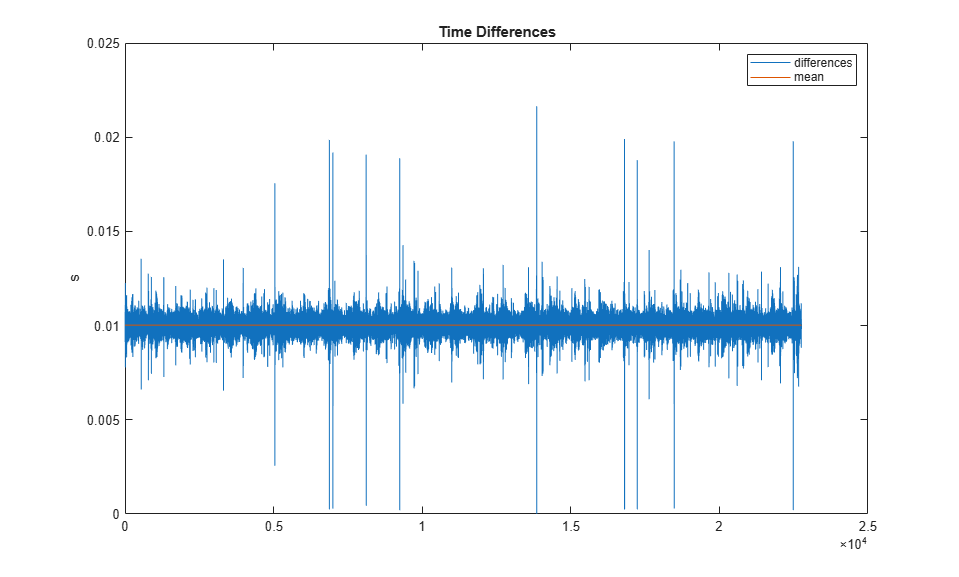### Compare the Transformed Logged Quaternion to the `imufilter` Quaternion

Conjugate the logged orientation quaternion before comparing it to the estimated orientation quaternion from `imufilter`. From the plot below, there is still a constant offset in the z-axis Euler angle estimate. This is because the `imufilter` assumes the initial orientation of the device is aligned with the navigation frame.

```loggedOrient = conj(orient); filt = imufilter('SampleRate', sampleRate); estOrient = filt(accel, gyro); figure subplot(2, 1, 1) plot(time, eulerd(loggedOrient, 'ZYX', 'frame'), '--') title('Logged Euler Angles') ylabel('\circ') % Degrees symbol. legend('z-axis', 'y-axis', 'x-axis') subplot(2, 1, 2) plot(time, eulerd(estOrient, 'ZYX', 'frame')) title('|imufilter| Euler Angles') ylabel('\circ') % Degrees symbol. legend('z-axis', 'y-axis', 'x-axis') ```### Align the Logged Orientation and `imufilter` Orientation

Align the `imufilter` orientation quaternion with the logged orientation quaternion by applying a constant bias using the first logged orientation quaternion. For quaternions, a constant rotation bias can be applied by pre-multiplying frame rotations or post-multiplying point rotations. Since `imufilter` reports quaternions as frame rotation operators, the estimated orientation quaternions are pre-multiplied by the first logged orientation quaternion.

```alignedEstOrient = loggedOrient(1) .* estOrient; figure subplot(2, 1, 1) plot(time, eulerd(loggedOrient, 'ZYX', 'frame'), '--') title('Logged Euler Angles') ylabel('\circ') % Degrees symbol. legend('z-axis', 'y-axis', 'x-axis') subplot(2, 1, 2) plot(time, eulerd(alignedEstOrient, 'ZYX', 'frame')) title('Aligned |imufilter| Euler Angles') ylabel('\circ') % Degrees symbol. legend('z-axis', 'y-axis', 'x-axis') ```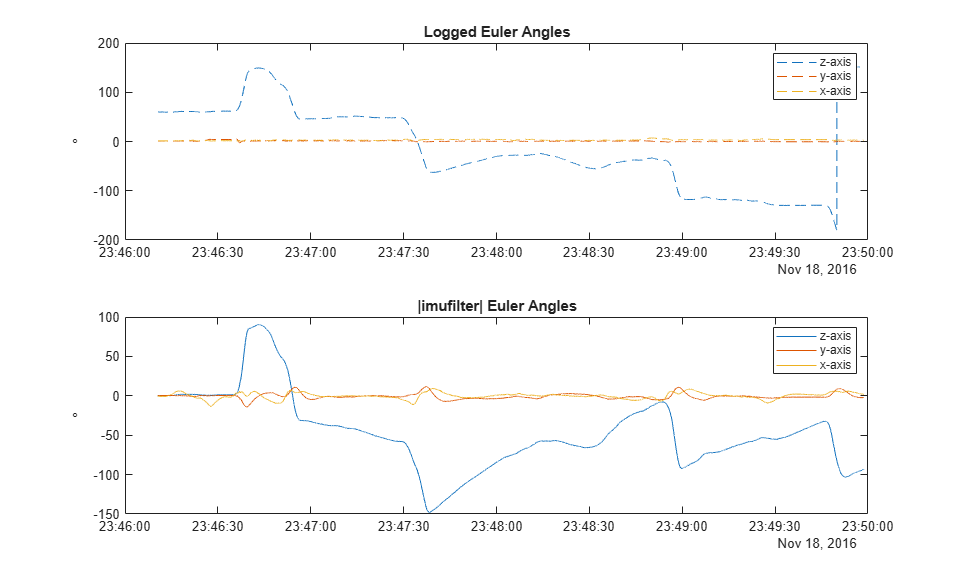### Conclusion

For the MAT-file in this example, you checked the following aspects for alignment:

• Units for accelerometer and gyroscope.

• Axes alignments of accelerometer and gyroscope.

• Orientation quaternion rotation operator (point:or frame:)

Different unit conversions, axes alignments, and quaternion transformations may need to be applied depending on the format of the logged data.